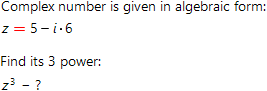# Find power of complex number online with step by step solutionOur online calculator allows one to find power of complex number with step by step solution. To use the calculator one should choose representation form of complex number (algebraic, trigonometric or exponential) and enter corresponding data. Below we give some basic knowledge of complex numbers.

Suppose we have complex number

z=x+iy

To find its nZ power, one need to calculate expression:

zn=(x+iy)n

To accomplish this task, one can use short multiplication formulas. For example:

z2=(x+iy)2= x2+2xiy+(iy)2= x2+2xyi-y2

However, for high values of n it would be simplier to use de Moivre's formula:

zn=rn∙(cos(nφ)+isin(nφ))

As expected, to use this formula, complex number should be given in the trigonometric form.

Finally, the simplest way to find power of complex number is to use exponential representation:

z=reiφ

Then:

zn=rneinφ

Power of complex number calculator